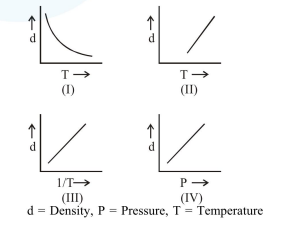# Which one of the following graphs is not correct for ideal gas?

Question:

Which one of the following graphs is not correct for ideal gas?1. II

2.  III

3. I

4. IV

Correct Option: 1

Solution:

$\mathrm{PM}=\mathrm{dRT} \Rightarrow \mathrm{d} \propto \frac{1}{\mathrm{~T}}$